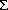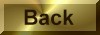Practice Problem 6

CalculateHḞ andSḞ   for the following reaction:

NH4NO3(s)  + H2O(lNH4+ (aq) + NO3- (aq)

Use the results of this calculation to determine the value ofGo for this reaction at 25o C, and explain why NH4NO3 spontaneously dissolves is water at room temperature.

Solution

Using a standard-state enthalpy of formation and absolute entropy data table, we find the following information:

CompoundHfo(kJ/mol)         SḞ(J/mol-K)

NH4NO3(s)                 -365.56             151.08

NH4+ (aq)                 -132.51               113.4

NO3- (aq)                 -205.0                 146.4

This reaction is endothermic, and the enthalpy of reaction is therefore unfavorable:Ho =Hfo(products) -Hfo(reactants)

= [1 mol NH4 x 132.51 kJ/mol + 1 mol NO3-  x -205.0 kJ/mol] - [1 mol NH4NO3 x -365.56 kJ/mol]

= 28.05 kJ

The reaction leads to a significant increase in the disorder of the system, however, and is therefore favored by the entropy of reaction:So =So(products) -So(reactants)

= [1 mol NH4 x 113.4 J/mol-K + 1 mol NO3-  x 146.4 J/mol-K] - [1 mol NH4NO3 x 151.08 J/mol-K]

= 108.7 J/K

To decide whether NH4NO3 should dissolve in water at 25o C we have to compare theHo and TSo to see which is larger.  Before we can do this, we have to convert the temperature from oC to kelvin:

TK = 25o C + 273.15 = 298.15 K

We also have to recognize that the units ofHo for this reaction are kilojoules and the units ofSo are joules per kelvin.  At some point in this calculation, we therefore have to convert these quantites to a consistent set of untis.  Perhaps the easiest way of doing this is to convertHo to joules.  We then multiply the entropy term by the absolute temperature and subtract this quantity from the enthalpy term:Go =Ho - TSo

= 28,050 J - (298.15 K x 108.7 J/K)

= 28,050 J - 32,410 J

= -4360 J

At 25o C, the standard-state free energy for this reaction is negative because the entropy term at this temperature is larger that the enthalpy term:Go = -4.4 kJ

The reaction is therefore spontaneous at room temperature.